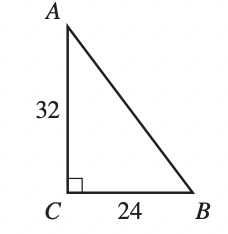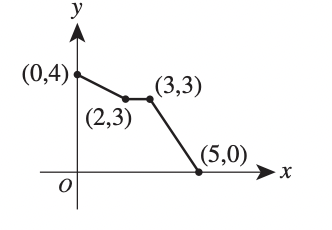# ACT Mathematics: Expression and Variables Equation Quiz

This is an MCQ-based quiz on the topic of ACT Mathematics: Expression and Variables Equation Quiz.

This set of MCQs helps you brush up on the important mathematics topic and prepare you to dive into skill practice and expand your knowledge to tackle the question and answer it carefully.

Start Quiz

The list of numbers 41, 35, 30, X, Y, 15 has a median of 25. The mode of the list of numbers is 15. To the nearest whole number, what is the mean of the list?

20

25

26

30

What is the value of the expression below?

⎪⎪−8 + 4⎪ − ⎪3 − 9⎪⎪

-18

-2

0

2

. What is the sum of the solutions of the 2 equations below?

8x = 12

2y + 10 = 22

12/5

15/2

9

10

A wallet containing 5 five-dollar bills, 7 ten-dollar bills, and 8 twenty-dollar bills is found and returned to its owner. The wallet’s owner will reward the finder with 1 bill drawn randomly from the wallet. What is the probability that the bill drawn will be a twentydollar bill?

1/20

4/51

1/8

2/5

. In the standard (x,y) coordinate plane, what is the midpoint of the line segment that has endpoints (3,8) and (1,−4) ?

-2, -12

-1, -6

11/2, -3/2

2,2

The length of a rectangle is 5 inches longer than the width. The perimeter of the rectangle is 40 inches. What is the width of the rectangle, in inches?

7.5

8

15

16

The lengths of the 2 legs of right triangle nABC shown below are given in inches. The midpoint of AB ___ is how many inches from A ?16

20

21

28

A box in the shape of a cube has an interior side length of 18 inches and is used to ship a right circular cylinder with a radius of 6 inches and a height of 12 inches. The interior of the box not occupied by the cylinder is filled with packing material. Which of the following numerical expressions gives the number of cubic inches of the box filled with packing material?

15 - pi

15 + pi

18^3 - pi*6*12*6

pi^3

The graph of a function y = f (x) consists of 3 line segments. The graph and the coordinates of the endpoints of the 3 line segments are shown in the standard (x,y) coordinate plane below. What is the area, in square coordinate units, of the region bounded by the graph of y = f(x), the positive y-axis, and the positive x-axis?10

13

14

15

The sum of 2 positive numbers is 151. The lesser number is 19 more than the square root of the greater number. What is the value of the greater number minus the lesser number?

19

66

85

91

Quiz/Test Summary
Title: ACT Mathematics: Expression and Variables Equation Quiz
Questions: 10
Contributed by: